# MCQs on Control Systems

##### Page 19 of 23. Go to page 1 2 3 4 5 6 7 8 9 10 11 12 13 14 15 16 17 18 19 20 21 22 23
01․ The closed loop transfer function of a unity feedback control system is 9/(s² + 3s + 9), then the type of the system is
3
0
2
1

Every transfer function representing a control system has certain type and order. The steady state analysis depends on the type of the control system. The type of the system is obtained from open loop transfer function. The number of open loop poles occurring at origin determines the type of the control system Open loop transfer function = 9/(s² + 3s + 9 - 9) = 9/s(s+3) Therefore, the type of the given system is 1.

02․ The closed loop transfer function of a system is 25/(s² + 25). The settling time of the system is
5 sec
1 sec
∞ sec
25 sec

Given system is marginally stable system. Therefore, the damping factor ξ is zero. Settling time = 4/(ξωn) Settling time = ∞ sec For given system response is oscillatory, which never settles.

increases the damping ratio
decreases the damping ratio
increases the band width
increases the stability

Lag compensator characteristics: 1. For sinusoidal input, the phase of controller output lags by tan-1 (1/ωTi). 2. In terms of filtering property it acts as low pass filter. 3. It increases rise time. 4. It decreases band width. 5. It decreases stability of the system. 6.The integral controller eliminates the offset of proportional controller. 7. Increases the damping ratio.

04․ Negative feedback in a closed loop control system
reduces gain.
improves disturbance rejection.
reduces sensitivity to parameter variation.
all of the above.

Advantages of closed loop control system:
1. 1. The closed loop systems are accurate
2. 2. The closed loop systems are accurate even in the presence of non linearities
3. 3. The sensitivity of the system may be made small to make the system more stable
4. 4. The closed loop systems are less affected by noise
Disadvantages of closed loop control system:
1. 1. The closed loop systems are complex and costly
2. 2. The feedback in closed loop system may lead to oscillatory response
3. 3. The feedback reduces the overall gain of the system
4. 4. Stability is the major problem in closed loop system and more care is needed to design a stable closed loop system.

05․ The characteristic equation for closed loop system is given as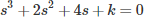. The value of k for which the system becomes just stable is
2
4
6
8

Given characteristic equation isApplying Routh-Hurwitz criterion as shown below,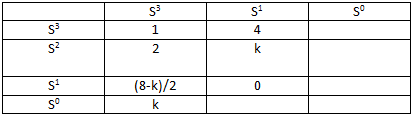For system becomes just stable, (8-k)/2 = 0 . Therefore, k = 8.

06․ The open loop transfer function of a unity feedback control system is G(s) = 1/((s(s+0.1s)(s+1)). The value of damping factor for which the resonant peek is equal to 1.4 is
0.386
0.263
0.953
0.707

Resonant peak = 1/(2ξ√(1-ξ²)) Given resonant peak = 1.4 1.4 = 1/(2ξ√(1-ξ²)) Therefore, damping factor ξ = 0.386

07․ The open loop transfer function of non unity negative feedback system is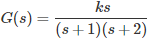, then the number of root locus branches equal to
0.
1.
2.
3.

The root locus is defined as the locus of closed loop poles obtained when system gain k is varied from 0 to ∞. The root locus is symmetrical bout real axis. Let P = Number of open loop poles and Z = Number of open loop zeros and P>Z. The number of branches of root locus = P The number of branches terminating at zeros = Z The number of branches terminating at infinity = P – Z

08․ A transfer function is given as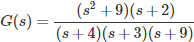The steady state sinusoidal response will become zero at a frequency response of

Given transfer function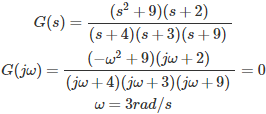09․ In frequency response analysis of second order system, the error at corner frequency is
20 log2ξ
10 logξ
-20 log2ξ
-10 logξ

In frequency response analysis of second order system, the magnitude in db value is |F(jω)| = -20 log√((1-(ω/ωn)²)²+(2ξω/ωn)²) Where ωn = Natural frequency At corner frequency ωcf = ω = ωn |F(jω)| = -20 log2ξ

10․ Which of the following represents the value of resonant frequency (ωr)?
n) √(1-ζ²)
n) √(1-2ζ²)
2*(ωn) √(1-ζ²)
n) √(1-ζ²/2)

Resonant frequency is the frequency at which magnitude has maximum value. Resonant frequency (ωr) = (ωn) √(1-2ζ²)

<<<1718192021>>>